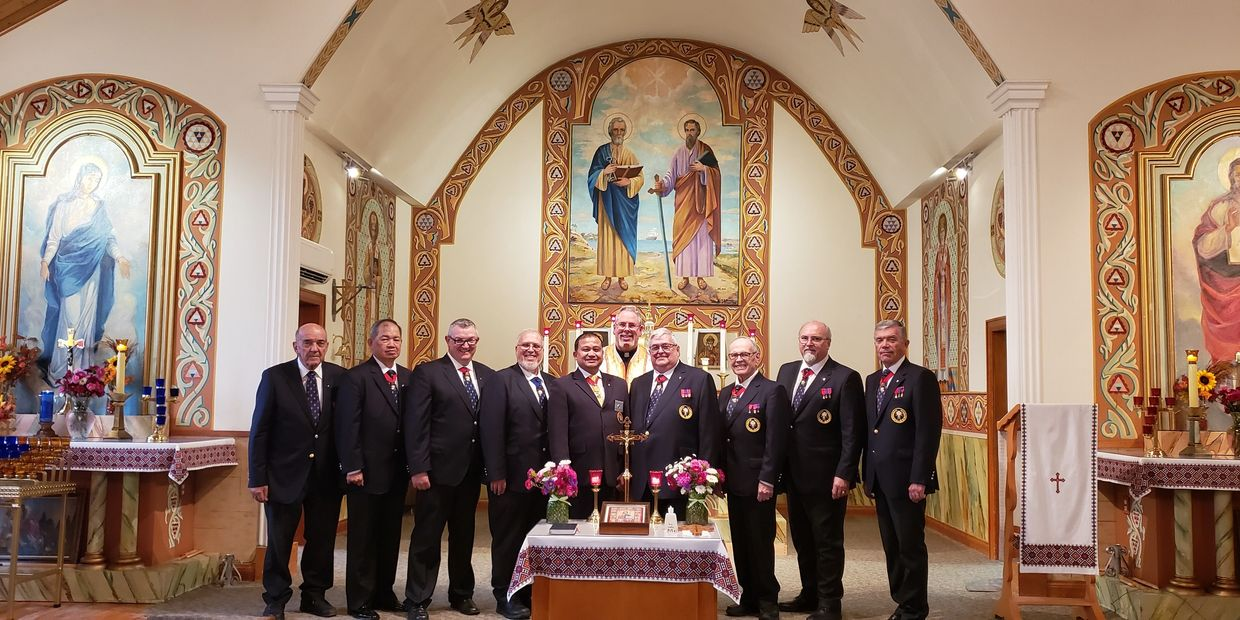# 5th Ontario District

4th Degree 4th Degree 4th Degree 4th Degree 4th Degree 4th Degree 4th Degree 4th Degree 4th Degree 4th Degree 4th Degree 4th Degree 4th Degree 4th Degree 4th Degree 4th Degree 4th Degree 4th Degree 4th Degree 4th Degree 4th Degree 4th Degree 4th Degree 4th Degree 4th Degree 4th Degree 4th Degree 4th Degree 4th Degree 4th Degree 4th Degree 4th Degree 4th Degree 4th Degree 4th Degree 4th Degree 4th Degree 4th Degree 4th Degree 4th Degree 4th Degree 4th Degree 4th Degree 4th Degree 4th Degree 4th Degree 4th Degree 4th Degree 4th Degree 4th Degree 4th Degree 4th Degree 4th Degree 4th Degree 4th Degree 4th Degree 4th Degree 4th Degree 4th Degree 4th Degree 4th Degree 4th Degree 4th Degree 4th Degree 4th Degree 4th Degree 4th Degree 4th Degree 4th Degree 4th Degree 4th Degree 4th Degree 4th Degree 4th Degree 4th Degree 4th Degree 4th Degree 4th Degree 4th Degree 4th Degree 4th Degree 4th Degree 4th Degree 4th Degree 4th Degree 4th Degree 4th Degree 4th Degree 4th Degree 4th Degree 4th Degree 4th Degree 4th Degree 4th Degree 4th Degree 4th Degree 4th Degree 4th Degree 4th Degree 4th Degree 4th Degree 4th Degree 4th Degree 4th Degree 4th Degree 4th Degree 4th Degree 4th Degree 4th Degree 4th Degree 4th Degree 4th Degree 4th Degree 4th Degree 4th Degree 4th Degree 4th Degree 4th Degree 4th Degree 4th Degree 4th Degree 4th Degree 4th Degree 4th Degree 4th Degree 4th Degree 4th Degree 4th Degree 4th Degree 4th Degree 4th Degree 4th Degree 4th Degree 4th Degree 4th Degree 4th Degree 4th Degree 4th Degree 4th Degree 4th Degree 4th Degree 4th Degree 4th Degree 4th Degree 4th Degree 4th Degree

# Knights of ColumbusCatholics defending our faithService to God & Country

Charity, Unity, Fraternity and Patriotism.

Vivat Jesus!

4th Degree 4th Degree 4th Degree 4th Degree 4th Degree 4th Degree 4th Degree 4th Degree 4th Degree 4th Degree 4th Degree 4th Degree 4th Degree 4th Degree 4th Degree 4th Degree 4th Degree 4th Degree 4th Degree 4th Degree 4th Degree 4th Degree 4th Degree 4th Degree 4th Degree 4th Degree 4th Degree 4th Degree 4th Degree 4th Degree 4th Degree 4th Degree 4th Degree 4th Degree 4th Degree 4th Degree 4th Degree 4th Degree 4th Degree 4th Degree 4th Degree 4th Degree 4th Degree 4th Degree 4th Degree 4th Degree 4th Degree 4th Degree 4th Degree 4th Degree 4th Degree 4th Degree 4th Degree 4th Degree 4th Degree 4th Degree 4th Degree 4th Degree 4th Degree 4th Degree 4th Degree 4th Degree 4th Degree 4th Degree 4th Degree 4th Degree 4th Degree 4th Degree 4th Degree 4th Degree 4th Degree 4th Degree 4th Degree 4th Degree 4th Degree 4th Degree 4th Degree 4th Degree 4th Degree 4th Degree 4th Degree 4th Degree 4th Degree 4th Degree 4th Degree 4th Degree 4th Degree 4th Degree 4th Degree 4th Degree 4th Degree 4th Degree 4th Degree 4th Degree 4th Degree 4th Degree 4th Degree 4th Degree 4th Degree 4th Degree 4th Degree 4th Degree 4th Degree 4th Degree 4th Degree 4th Degree 4th Degree 4th Degree 4th Degree 4th Degree 4th Degree 4th Degree 4th Degree 4th Degree 4th Degree 4th Degree 4th Degree 4th Degree 4th Degree 4th Degree 4th Degree 4th Degree 4th Degree 4th Degree 4th Degree 4th Degree 4th Degree 4th Degree 4th Degree 4th Degree 4th Degree 4th Degree 4th Degree 4th Degree 4th Degree 4th Degree 4th Degree 4th Degree 4th Degree 4th Degree 4th Degree 4th Degree 4th Degree 4th Degree 4th Degree 4th Degree

# Knights of ColumbusCatholics defending our faithService to God & Country

Charity, Unity, Fraternity and Patriotism.

Vivat Jesus!

## FVSM & VSM and Masters of Cardinal McGuigan Province

#### Cardinal mcguigan provincePhoto left to right

Marcel Wozney FVSM

Rudy Montaniel DM 2

Daniel Mainville DM 3

Karl Brosch VSM

David Peters State Deputy

Fr. Darren Kawiuk

Denis Amyotte DM 6

Rene Chabot DM 7

Dan Lohin DM 8

Reg Campbell DM 5

Men of Faith. Faith in Action.

Website updated Oct 10th, 2019